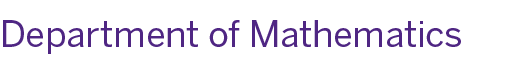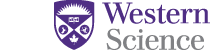# 2019-20 Course List (Tentative)

Tentative Fall/Winter 2019-20 Courses in Calculus and Mathematics

• Calculus 1000A: Calculus I
• Calculus 1000B: Calculus I (offered by the Department of Applied Mathematics)
• Calculus 1500A: Calculus I for the Mathematical Sciences
• Calculus 1301B: Calculus II (offered by the Department of Applied Mathematics)
• Calculus 1501B: Calculus II for Mathematical and Physical Sciences
• Calculus 2302A: Intermediate Calculus I
• Calculus 2303B: Intermediate Calculus II (offered by the Department of Applied Mathematics)
• Calculus 2402A: Calculus with Analysis for Statistics (offered by the Department of Applied Mathematics)
• Calculus 2502A: Advanced Calculus I
• Calculus 2503B: Advanced Calculus II (offered by the Department of Applied Mathematics)
• Mathematics 0110A: Introductory Calculus
• Mathematics 1120B: Fundamental Concepts in Mathematics
• Mathematics 1225B: Methods of Calculus
• Mathematics 1228A: Methods of Finite Mathematics
• Mathematics 1228B: Methods of Finite Mathematics
• Mathematics 1229A: Methods of Matrix Algebra
• Mathematics 1229B: Methods of Matrix Algebra
• Mathematics 1600A: Linear Algebra I
• Mathematics 1600B: Linear Algebra I
• Mathematics 2120B: Intermediate Linear Algebra
• Mathematics 2122B:Real Analysis I
• Mathematics 2124A: Introduction to Mathematical Problems
• Mathematics 2151A: Discrete Structures for Engineering
• Mathematics 2155F: Mathematical Structures
• Mathematics 3020A: Introduction to Abstract Algebra
• Mathematics 3120B: Group Theory
• Mathematics 3122A: Real Analysis II
• Mathematics 3124A: Complex Analysis I
• Mathematics 3152B: Combinatorics
• Mathematics 3157B: Game Theory
• Mathematics 4122B: Introduction to Measure Theory
• Mathematics 4123A: Rings and Modules
• Mathematics 4151B: Algebraic Number Theory
• Mathematics 4152A: Algebraic Topology
• Mathematics 4153B: Algebraic Geometry
• Mathematics 4154A: Introduction to Functional Analysis
• Mathematics 4156B: Complex Analysis

Fall/Winter 2019-20 Recommended Courses Offered by Other Departments (Tentative)

• Applied Mathematics 2402A: Ordinary Differential Equations
• Applied Mathematics 2814G: Numerical Analysis
• Applied Mathematics 3815A: Partial Differential Equations I
• Computer Science 1026A/B: Computer Science Fundamentals I
• Computer Science 1027A/B: Computer Science Fundamentals II
• Statistical Sciences 2857A: Probability and Statistics I
• Statistical Sciences 2858B: Probability and Statistics II
• Statistical Sciences 3857A: Intermediate Probability